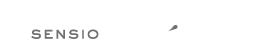Fork me on GitHub# Rule `modernize_types_casting`¶

Replaces `intval`, `floatval`, `doubleval`, `strval` and `boolval` function calls with according type casting operator.

## Warning¶

### Using this rule is risky¶

Risky if any of the functions `intval`, `floatval`, `doubleval`, `strval` or `boolval` are overridden.

## Examples¶

### Example #1¶

```--- Original
+++ New
<?php
-    \$a = intval(\$b);
-    \$a = floatval(\$b);
-    \$a = doubleval(\$b);
-    \$a = strval (\$b);
-    \$a = boolval(\$b);
+    \$a = (int) \$b;
+    \$a = (float) \$b;
+    \$a = (float) \$b;
+    \$a = (string) \$b;
+    \$a = (bool) \$b;
```

## Rule sets¶

The rule is part of the following rule sets:

## Source class¶

PhpCsFixer\Fixer\CastNotation\ModernizeTypesCastingFixer